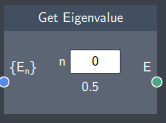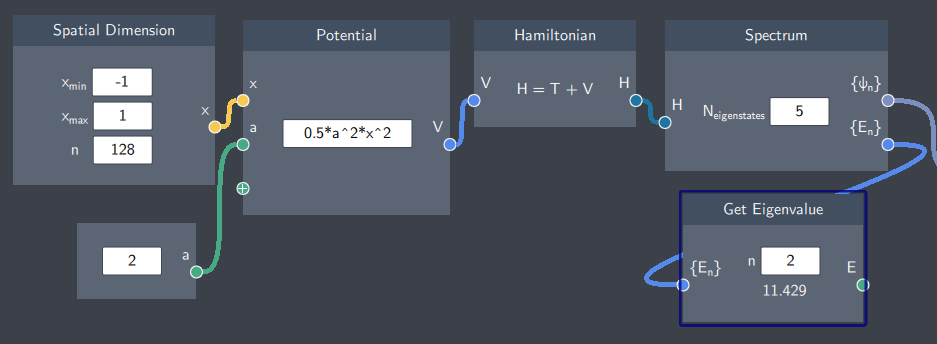# Get Eigenvalue

## Description

This node extracts a single eigenvalue from the spectrum of eigenvalues of the system defined by the Hamiltonian.## Input

The node has the following input:

• Spectrum of eigenvalues ($\{ E_n \}$): The list of eigenvalues of the system (defined by a Hamiltonian) calculated in the Spectrum node.

## Content

In the content (text) field, the index of the desired energy level is entered and the corresponding eigenvalue is displayed below the content (text) field. Note that the indexing starts with zero, which means that n=0 corresponds to the eigenvalue of the ground state. Moreover, the scalar in the $n$ field should be smaller to the scalar in the $N_{eigenstates}$ field ( in the Spectrum node) otherwise the desired eigenvalue is not in the spectrum of eigenvalues and the node will display an error message.

## Output

• Eigenvalue (E): The $n^{th}$ eigenvalue extracted from the energy spectrum. The eigenvalue is displayed in the node itself.

## Example

In the example below, the energy spectrum of a harmonic oscillator is calculated. The Spectrum node outputs the first 5 eigenvalues and inputs it in the Get Eigenvalue node.

As the text-field is set to "2" in the Get Eigenvalue node, the value of the second excited state (11.429)  is displayed in the node.